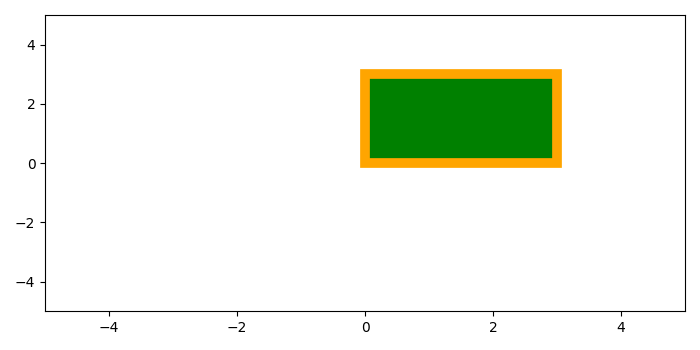# How do I set color to Rectangle in Matplotlib?

To set color to a rectangle in matplotlib, we can take the following steps −

• Create a figure or activate an existing figure using figure() method.

• Add an '~.axes.Axes' to the figure as part of a subplot arrangement using add_subplot() method.

• A rectangle is defined via an anchor point with width and heights.

• Add a rectangle patch to the plot.

• Set the x and y limit using xlim() and ylim() method.

• To display the figure, use show() method.

## Example

from matplotlib import pyplot as plt, patches
plt.rcParams["figure.figsize"] = [7.00, 3.50]
plt.rcParams["figure.autolayout"] = True
fig = plt.figure()
plt.show()